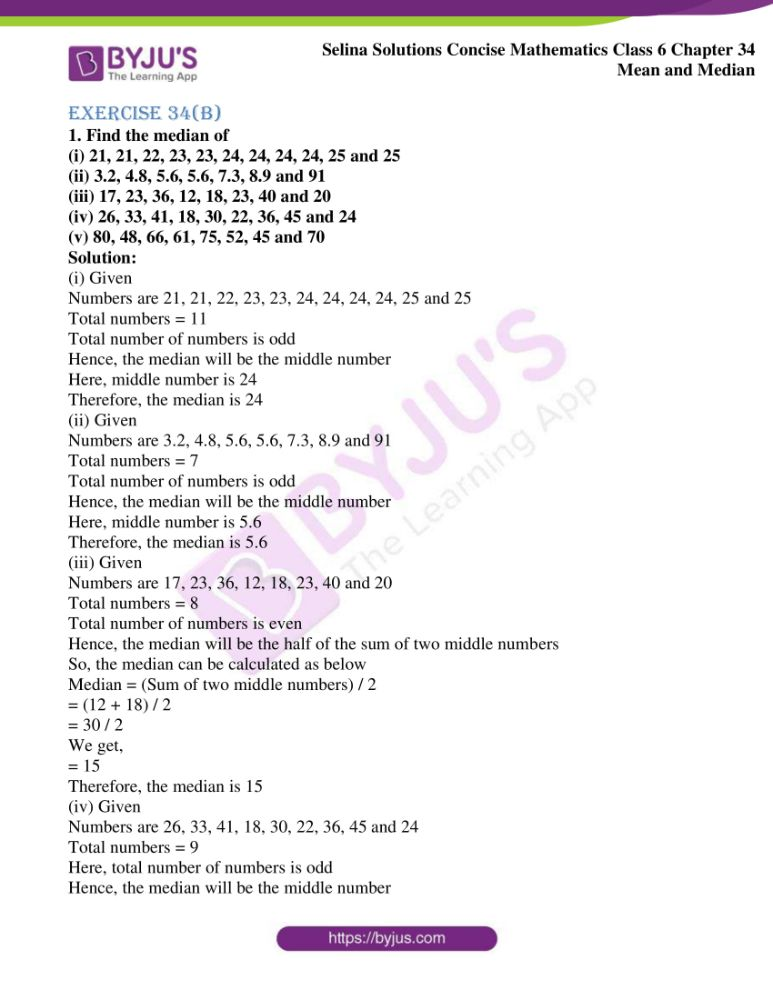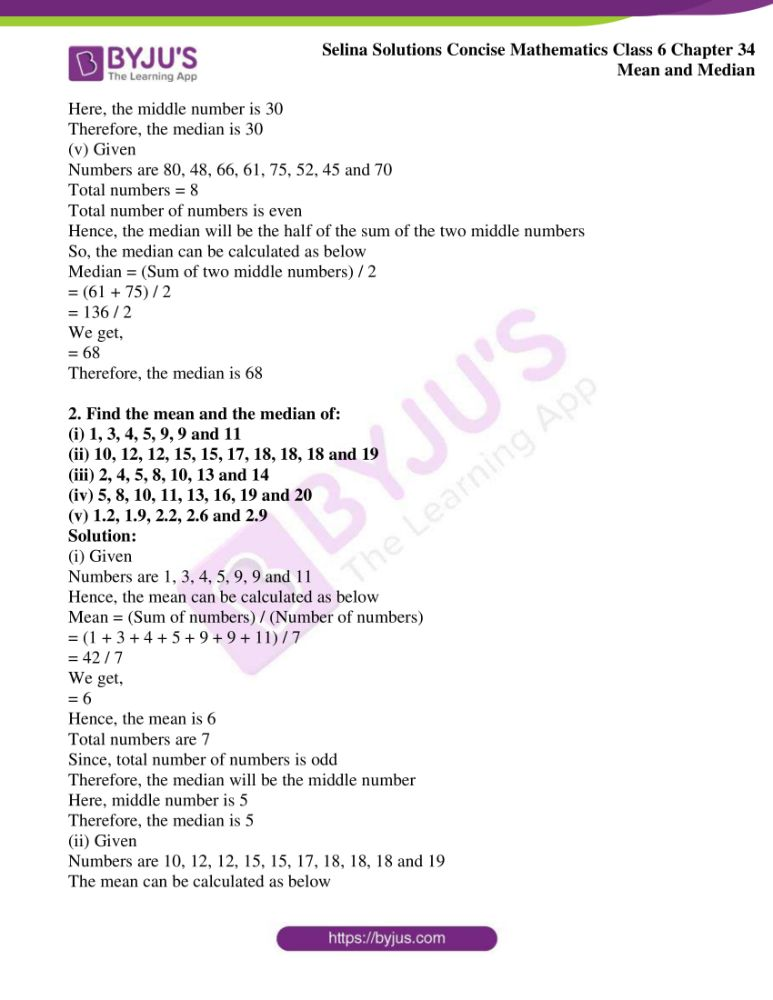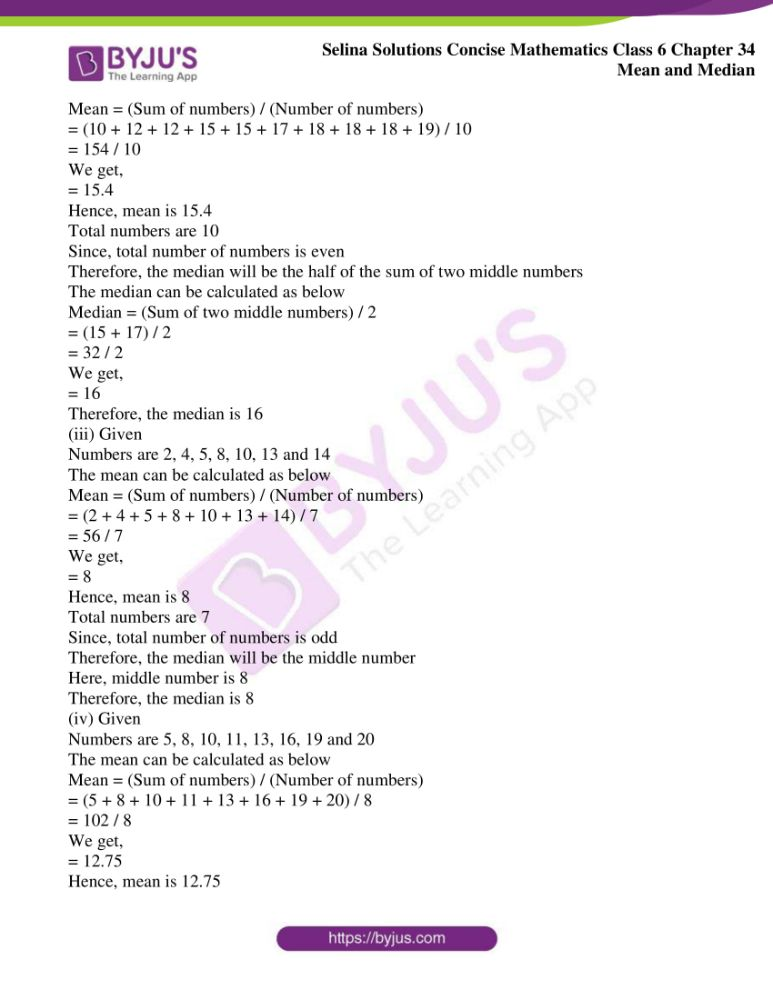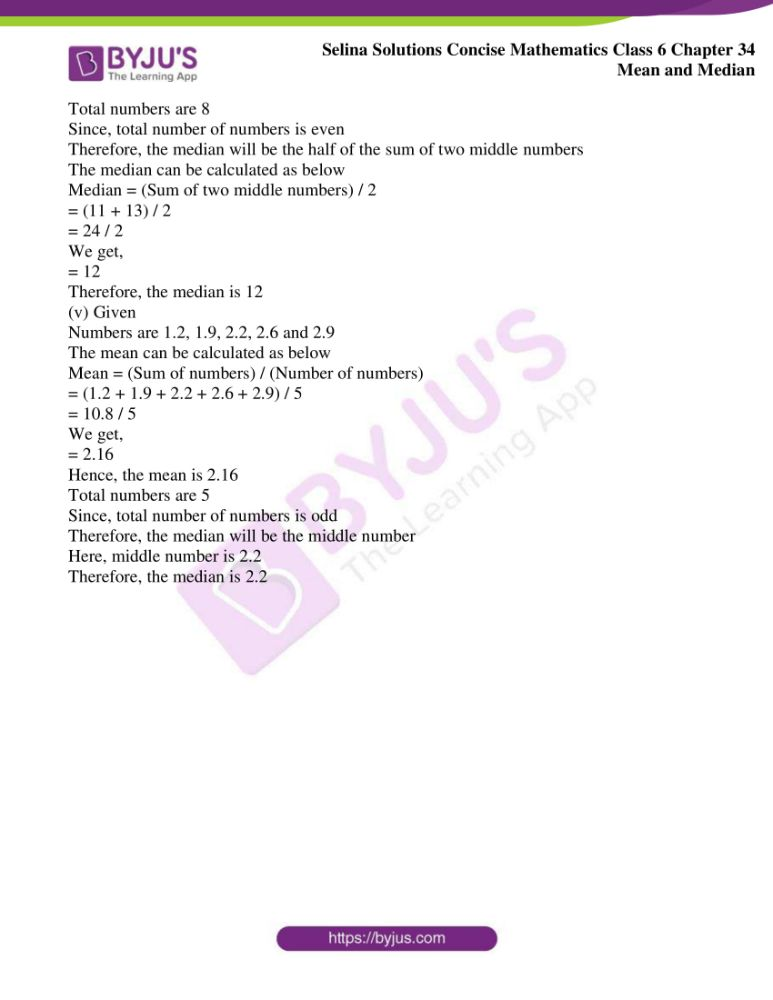# Selina Solutions Concise Mathematics Class 6 Chapter 34 Mean and Median Exercise 34(B)

Selina Solutions Concise Mathematics Class 6 Chapter 34 Mean and Median Exercise 34(B) are made available in PDF format, as per the students’ needs. These solutions mainly improve analytical and logical thinking among students to solve complex problems with ease. The solutions also provide important shortcut methods of solving problems in a shorter duration. Continuous practice of these solutions, help students to come out with flying colours in their academics. To get their doubts cleared instantly, students can access Selina Solutions Concise Mathematics Class 6 Chapter 34 Mean and Median Exercise 34(B) PDF, from the links which are available here.

## Selina Solutions Concise Mathematics Class 6 Chapter 34 Mean and Median Exercise 34(B) Download PDF### Access Selina Solutions Concise Mathematics Class 6 Chapter 34 Mean and Median Exercise 34(B)

Exercise 34(B)

1. Find the median of

(i) 21, 21, 22, 23, 23, 24, 24, 24, 24, 25 and 25

(ii) 3.2, 4.8, 5.6, 5.6, 7.3, 8.9 and 91

(iii) 17, 23, 36, 12, 18, 23, 40 and 20

(iv) 26, 33, 41, 18, 30, 22, 36, 45 and 24

(v) 80, 48, 66, 61, 75, 52, 45 and 70

Solution:

(i) Given

Numbers are 21, 21, 22, 23, 23, 24, 24, 24, 24, 25 and 25

Total numbers = 11

Total number of numbers is odd

Hence, the median will be the middle number

Here, middle number is 24

Therefore, the median is 24

(ii) Given

Numbers are 3.2, 4.8, 5.6, 5.6, 7.3, 8.9 and 91

Total numbers = 7

Total number of numbers is odd

Hence, the median will be the middle number

Here, middle number is 5.6

Therefore, the median is 5.6

(iii) Given

Numbers are 17, 23, 36, 12, 18, 23, 40 and 20

Total numbers = 8

Total number of numbers is even

Hence, the median will be the half of the sum of two middle numbers

So, the median can be calculated as below

Median = (Sum of two middle numbers) / 2

= (12 + 18) / 2

= 30 / 2

We get,

= 15

Therefore, the median is 15

(iv) Given

Numbers are 26, 33, 41, 18, 30, 22, 36, 45 and 24

Total numbers = 9

Here, total number of numbers is odd

Hence, the median will be the middle number

Here, the middle number is 30

Therefore, the median is 30

(v) Given

Numbers are 80, 48, 66, 61, 75, 52, 45 and 70

Total numbers = 8

Total number of numbers is even

Hence, the median will be the half of the sum of the two middle numbers

So, the median can be calculated as below

Median = (Sum of two middle numbers) / 2

= (61 + 75) / 2

= 136 / 2

We get,

= 68

Therefore, the median is 68

2. Find the mean and the median of:

(i) 1, 3, 4, 5, 9, 9 and 11

(ii) 10, 12, 12, 15, 15, 17, 18, 18, 18 and 19

(iii) 2, 4, 5, 8, 10, 13 and 14

(iv) 5, 8, 10, 11, 13, 16, 19 and 20

(v) 1.2, 1.9, 2.2, 2.6 and 2.9

Solution:

(i) Given

Numbers are 1, 3, 4, 5, 9, 9 and 11

Hence, the mean can be calculated as below

Mean = (Sum of numbers) / (Number of numbers)

= (1 + 3 + 4 + 5 + 9 + 9 + 11) / 7

= 42 / 7

We get,

= 6

Hence, the mean is 6

Total numbers are 7

Since, total number of numbers is odd

Therefore, the median will be the middle number

Here, middle number is 5

Therefore, the median is 5

(ii) Given

Numbers are 10, 12, 12, 15, 15, 17, 18, 18, 18 and 19

The mean can be calculated as below

Mean = (Sum of numbers) / (Number of numbers)

= (10 + 12 + 12 + 15 + 15 + 17 + 18 + 18 + 18 + 19) / 10

= 154 / 10

We get,

= 15.4

Hence, mean is 15.4

Total numbers are 10

Since, total number of numbers is even

Therefore, the median will be the half of the sum of two middle numbers

The median can be calculated as below

Median = (Sum of two middle numbers) / 2

= (15 + 17) / 2

= 32 / 2

We get,

= 16

Therefore, the median is 16

(iii) Given

Numbers are 2, 4, 5, 8, 10, 13 and 14

The mean can be calculated as below

Mean = (Sum of numbers) / (Number of numbers)

= (2 + 4 + 5 + 8 + 10 + 13 + 14) / 7

= 56 / 7

We get,

= 8

Hence, mean is 8

Total numbers are 7

Since, total number of numbers is odd

Therefore, the median will be the middle number

Here, middle number is 8

Therefore, the median is 8

(iv) Given

Numbers are 5, 8, 10, 11, 13, 16, 19 and 20

The mean can be calculated as below

Mean = (Sum of numbers) / (Number of numbers)

= (5 + 8 + 10 + 11 + 13 + 16 + 19 + 20) / 8

= 102 / 8

We get,

= 12.75

Hence, mean is 12.75

Total numbers are 8

Since, total number of numbers is even

Therefore, the median will be the half of the sum of two middle numbers

The median can be calculated as below

Median = (Sum of two middle numbers) / 2

= (11 + 13) / 2

= 24 / 2

We get,

= 12

Therefore, the median is 12

(v) Given

Numbers are 1.2, 1.9, 2.2, 2.6 and 2.9

The mean can be calculated as below

Mean = (Sum of numbers) / (Number of numbers)

= (1.2 + 1.9 + 2.2 + 2.6 + 2.9) / 5

= 10.8 / 5

We get,

= 2.16

Hence, the mean is 2.16

Total numbers are 5

Since, total number of numbers is odd

Therefore, the median will be the middle number

Here, middle number is 2.2

Therefore, the median is 2.2